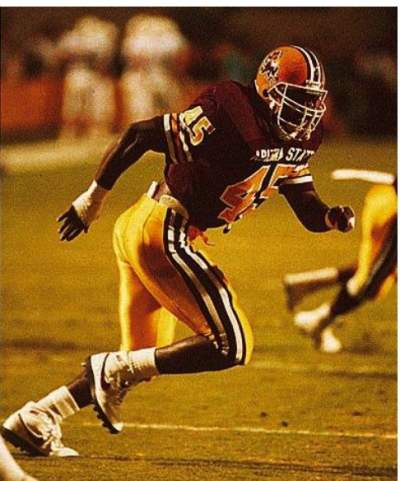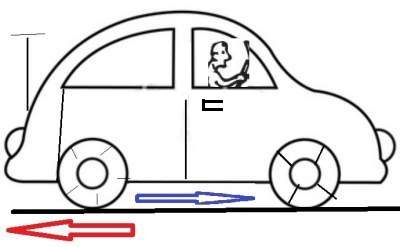# Newton's Third Law

## Defintion

For every action force there must be an equal and opposite reaction force.

Whenever there is an action force, there will be a reaction force against it.
For example, if we use a hammer to strike a nail, we are exerting an action force,
and the nail must also react back by pushing back against the hammer.

Newton’s third law states that these action forces and the opposite reaction forces are
equal in magnitude and opposite in directions.

Since action and reaction forces are equal and opposite, how come they DO NOT cancel out to zero?

These forces do not cancel each other because they are acting on two different bodies. See figure below:It is imperative to identify the forces acting on an object.
• The forces acting on the box are W (gravitational force from Earth) and N (normal force from the support table).
• Normal force refers to the perpendicular force a surface exerts on an object.
• The reaction force to the Earth’s attractive force W on the box, is an equal attractive force −W the box exerts on the Earth.
• The reaction force to the Earth’s attractive force W on the box, is an equal attractive force −W the box exerts on the Earth.
• The reaction force to the table’s normal force N exerted upward on the box, is an equal force −N the box exerts downward on the table.

## Practical examples of Newton's third law

### Example 1:

The football player's foot is pushing against the ground (see photo below), in return the ground pushes against the foot that accelerates the player forward.### Example 2:

Action: Car through its tires push on the road.
Reaction: Road pushes on tires (see below):### Exercises

Exercise 1 on Newton's Third Law